American Journal of Applied Scientific Research
Volume 1, Issue 2, November 2015, Pages: 18-26

Analysis of Repeated Measures Data of Iraqi Awassi Lambs Using Mixed Model

Firas Rashad Al-Samarai1, Fatten Ahmed Mohammed2, Falah Hamed Al-Zaidi2, Abbas Fawzy Al-Kalisy1

1Department of Veterinary Public Health, College of Veterinary Medicine, University of Baghdad, Baghdad, Iraq

2Department of Veterinary and Animal Resource, Directorate of Baghdad Agriculture, Ministry of Agriculture, Baghdad, Iraq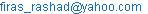(F. R. Al-Samarai)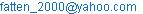(F. A. Mohammed)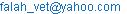(F. H. Al-Zaidi)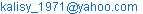(A. F. Al-Kalisy)

Firas Rashad Al-Samarai, Fatten Ahmed Mohammed, Falah Hamed Al-Zaidi, Abbas Fawzy Al-Kalisy. Analysis of Repeated Measures Data of Iraqi Awassi Lambs Using Mixed Model.American Journal of Applied Scientific Research.Vol.1, No. 2, 2015, pp. 18-26. doi: 10.11648/j.ajasr.20150102.13

Abstract: In this study, repeated records of body-weight of Awassi lambs were considered for analysis. Records included up to five ‘repeated records’ of body-weight per lamb, measured between birth weight and 4th month of age, were used in the analysis. Most statistical approaches in such data are based on analysis of variance (ANOVA). However, the assumption that datum are independent is usually violated since several measures are performed on the same subject. As a result, standard regression and ANOVA may produce invalid results of repeated measures data because they require mathematical assumptions that were inconsistent with repeated data. The newest approach to analyzing of the repeated measurements is a mixed-model analysis. Advocates of this approach claimed that it provides the "best" approach to the analysis of repeated measurements. Therefore, the objective of the study was to investigate the effect of flock on growth performance of Awassi lambs using the mixed model. Three models was used: the first model consist of the effect of flock, time and flock by time interaction, the second model includes the same factors besides the quadratic effect of time, and the third model includes all factors in second model besides the time by time by flock interaction. Results revealed that the third model was better than other models and the effect of all factors on body weight of lambs was significant (P< 0.05) except the effect of flock, which was non-significant.

Keywords: Awassi, Growth Performance, Repeated ANOVA, Mixed Model

Contents

1. Introduction

Sheep are efficient converters of unutilized poor quality grass and crop residues into meat (Kebede and Gebretsadik 2010). The rise in demand along with the price of sheep meat increased the interesting of researchers in reproductive performance and growth rate of sheep. In animal growth studies, body weight measurements, which are good indicators of growth rate, is often measured in the same animal at various ages (time points) such as weekly/monthly resulting in longitudinal growth data (Ganesan et al., 2014). Most statistical approaches in experiments of such data are based on analysis of variance (ANOVA). However, the assumption that data are independent is usually violated since several measures are performed on the same subject (repeated measures). As a result, standard regression and ANOVA may produce invalid results of repeated measures data because they require mathematical assumptions that do not consistent with these data (Lal, 2010).

The repeated measures aspect of the data makes it interesting because observations on the same subject are usually correlated and often exhibit the heterogeneous variability. If such correlation and heterogeneity are not present, ANOVA is appropriate because it assumes the observations are uncorrelated and have constant variance. When these properties are present, another methodology that accounts for them must be used, especially with regards to inferences about the fixed effects. Both repeated ANOVA and Mixed model offer repeated measures analyze that account for within-subject covariability (Littell et al., 1998).

Two different kinds of tests are available for the within-subject effects: univariate and multivariate. The univariate tests are appropriate when the within-subject variance-covariance matrix of the observations has a certain structural form known as Type H (Huynh and Feldt, 1970). Sphericity test is a statistical test for this structure. The sphericity test could be used to indicate which is most appropriate: the MANOVA or the univariate.

The sphericity assumption states that the variance of the difference scores in a within-subjects design (the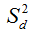in a paired t-test) are equal across all the groups. It is similar to the homogeneity of variance assumption with between subjects ANOVA. When this assumption is violated, there will be an increase in Type I errors because the critical values in the F-table are too small. There are several different approaches to correcting for this bias: Lower bound correction, Huynh and Feldt correction, Geisser-Greenhouse correction and Mauchly's test. When the sphericity test does not have a significant p-value, the univariate tests for within-subject effects must be used because under the Type H assumption they will usually be more powerful than the multivariate tests. When the sphericity test is significant, there are two ways to test the significance of the within-subject effects. (Greenhouse and Geisser 1959) and H-F (Huynh and Feldt 1976). The second way involves four different multivariate tests: Wilks’ Lambda, Pillai’s Trace, Hotelling-Lawley Trace, and Roy’s Greatest Root. These tests are all based on a completely general (unstructured) within-subject variance covariance matrix. Algina and Kesselman (1997) suggested that for few levels of the factor (i.e., time points) and large sample size, the MANOVA approach may be more powerful. Their recommendation is to use MANOVA if (a) the number of levels of the factor is less than or equal to 4 and the sample size is greater than the number of levels plus 15 or (b) the number of levels is between 5 and 8 and the sample size is greater than the number of levels plus 30. The newest approach to the analysis of repeated measurements that was adopted by several researchers (Littell et al., 1998; Akbaş et al., 2001; Eyduran and Akbas, 2010; Orhan et al., 2010) is a mixed-model analysis. Advocates of this approach claimed that it provides the "best" approach to the analysis of repeated measurements. Littell et al., (1996) stated that: when validity of the sphericity assumption is violated, mixed model methodology allow statisticians to specify different covariance structures for data with/without missing observations and were more superior to univariate. To support using the mixed model for analyzing repeated measurements, graphical presentation was performed to display the data with respect to its structure and the mode1 used for fitting the data. Hence, the aims of this study were to examine the growth performance using mixed model methodology by using three models along with graphical presentation to determine the most appropriate model for fitting the body weights of Awassi lambs over four months.

2. Materials and Methods

In the current work, 99 Awassi male lambs, single-born from dams at the age of 2-3 years, were randomly selected from three Awassi sheep flocks located in Abo-Gharib west of Baghdad /Iraq. All lambs were born during December, 2013.

Body weights of all lambs were measured at five times (0, 1, 2, 3, and 4 months).

Repeated measure design with two factors, flocks (between-subject) and time (within-subject) was used to fitting the data. Greenhouse-Geisser Epsilon (G-G) and Huynh-Feldt Epsilon (H-F) adjusted F test approaches were traditionally used when the sphericity assumption for univariate approach was violated (Keskin and Mendeş, 2001). Mixed model methodology was performed to analyze the data. Three statistical models were used for data analysis:

The first statistical model is:

Yijk = μ +αi + βj+ (αβ)ij+ eijk

Where:

μ= Grand mean

i=1, 2, 3(flocks) and j=0, 1 ….4 (time/month).

αi: i th fixed level of flock (treatment) factor

βk : kth fixed time effect,

(αβ)ik: Flock by time interaction effect,

eijk : Random error element.

The second model including the same factors in addition to time*time and the third model including the same factors in the second model in addition to time*time*flock.

Statistical analysis was carried out using GLM and MIXED procedures of SAS. Four criteria were estimated (-2Res. Log likelihood, AIC, AICC, and BIC) to determine the most suitable mixed model approach in repeated measures design. The lowest values of each criterion indicate the best covariance structure.

3. Results and Discussion

Least square means of body weight in three flocks are shown in Table (1) and presented in Figure (1). The Figure (1) illustrate that the flocks have lines that are not flat, i.e. the slopes of the lines are not equal to zero. The lines for the three flocks are rather far apart. Since the lines are not parallel, these could indicate that the interaction between time and flock is significant.

Results showed that the effects between subjects (flock), within the subject (time) and flock by time interaction were significant (Table 2, 3).

Table 1. Least square means (LSM) of the body weight of Awassi lambs for four months.

 Flock Birth weight Months 1 2 3 4 1 4.10±0.17 11.78±0.41a 17.85±0.58a 23.70±0.75a 28.33±0.97a 2 4.20±0.17 11.41±0.40a 16.85±0.58ab 21.70±0.75ab 25.83±0.97ab 3 3.91±0.15 10.14±0.35b 16.10±0.51b 20.71±0.65b 25.75±0.85b

LSM with different letters in the same column differ significantly (P < 0.05)

Table 2. Repeated ANOVA for between subjects effects.

 Source DF Type III SS Mean square F value P>F Flock 2 285.2187 142.6093 3.47 0.0350 Error 96 3942.4926 41.0676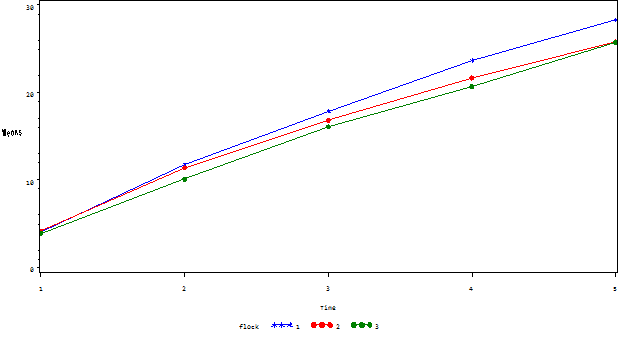Figure 1. Least square means of the body weight of Awassi lambs for four months.

Table 3. Repeated ANOVA for within subjects effects.

 Source DF Type III SS Mean square F value P>F Adj P>F G-G H-F Time 4 30874.1240 7718.5310 1528.22 <0.0001 <0.0001 <0.0001 Time*Flock 8 109.1157 13.6394 2.70 0.0067 0.0472 0.0472 Error 384 1939.4532 5.0506 Greenhouse-Geisser Epsilon 0.3799 Huynh-Feldt Epsilon 0.3927

In order to indicate which is most appropriate: the MANONA or the univariate test, sphericity test was performed and results revealed that the sphericity test (Table 4) was significant then hypothesis that the variance-covariance structure has a Type H structure was rejected. In such case, it is most appropriate to use the results from the MANOVA test.

Table 4. Test of sphericity.

 Variables DF Mauchly's criterion Chi-square P>F Transformed variables 9 0.00298 549.02 <0.0001 Orthogonal components 9 0.03839 307.78 <0.0001

Table 5. MANOVA test criteria for the hypothesis of no time effect.

 Statistics Value F value Num DF Den DF P>F Wilks' Lambda 0.043 515.33 4 93 <.0001 Pillai's Trace 0.956 515.33 4 93 <.0001 Hotelling-Lawley Trace 22.16 515.33 4 93 <.0001 Roy's Greatest Root 22.16 515.33 4 93 <.0001

MANOVA test criteria and exact F statistics for the hypothesis of no time effect and no time*flock effect was rejected at level of (P< 0.0001) and (P <0.003) respectively (Table 5, 6).

Table 6. MANOVA test criteria for the hypothesis of no time*flock effect.

 Statistics Value F value Num DF Den DF P>F Wilks' Lambda 0.771 3.22 8 186 <.0019 Pillai's Trace 0.236 3.15 8 188 <.0023 Hotelling-Lawley Trace 0.285 3.30 8 130.55 <.0018 Roy's Greatest Root 0.243 5.73 8 94 <.0004

Then the data subjected to the mixed model using proc mix in SAS program. The submitting of proc mixed in SAS program need to reshape the data from its wide form to a long form. To take a look at the distribution of data, scatter plot of data was performed with lines connecting the points for each individual as shown in Figure 2. The scatter plot offers a better understanding of the data.

The first mixed model used included time, flock and flock by time interaction. Figure (3) illustrate the predicted values of body weight of Awassi lambs and Figure (4) illustrates the predicted values plotted against the actual values of the lambs' body weight.Figure 2. Scatter plot of body weight of Awassi lambs with lines connecting the points for each individual.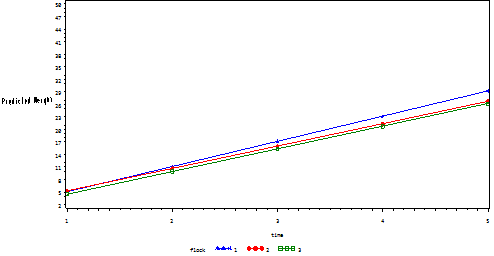Figure 3. The predicted values of the body weight of Awassi lambs according to the first model.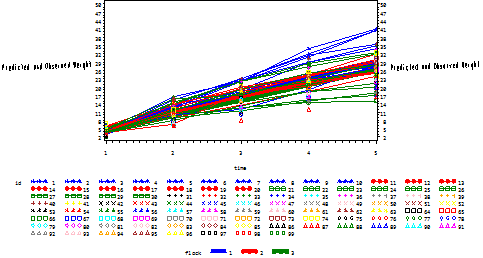Figure 4. Predicted values plotted against the actual values of body weight for the first model.

The values of the criteria are between 2118 and 2127 for model 1(Table 7). The effect of flock by time interaction was significant (P=0.02) (Table 8).

Table 7. Fitting criteria results for comparing three models.

 Statistics Model 1 Model 2 Model 3 -2Res. Log likelihood 2118.4 2014.9 2012.8 AIC 2122.4 2020.9 2018.8 AICC 2122.4 2020.9 2018.9 BIC 2127.6 2028.6 2026.6

Table 8. Type 3 tests of fixed effects (Model 1).

 Effect DF Den DF F value P> F Time 1 96 2608.20 <0.0001 Flock 2 297 3.42 0.0341 Time*Flock 2 297 3.71 0.0256

To model the quadratic effect of time, the factor time*time was added to the model and its effect was significant (P<0.0001) (Table 9). The values of the criteria are between 2014.9 and 2028.6 for model 1(Table 7). These results indicated that the second model was more fit to data as compared with the first model.

Table 9. Type 3 tests of fixed effects (Model 2).

 Effect DF Den DF F value P> F Time 1 96 1174.33 <0.0001 Flock 2 296 4.06 0.0183 Time*Flock 2 296 3.73 0.0251 Time*Time 1 96 130.42 <0.0001

The predicted values of body weight of Awassi lambs for the second model were shown in Figure 5. When the predicted values plotted against the actual values of body weight (Figure 6), the model fits better as the values of – 2 Res. Log, AIC, AICC, and BIC were lowered than the values of the same criteria in the first model (Table 7).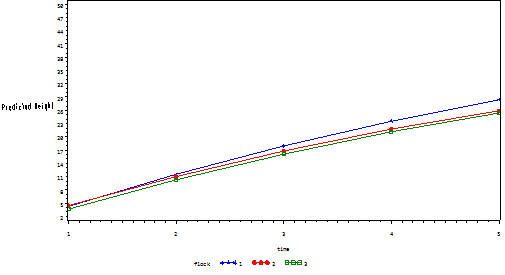Figure 5. Predicted values of the second model with the quadratic effect of time for the second model.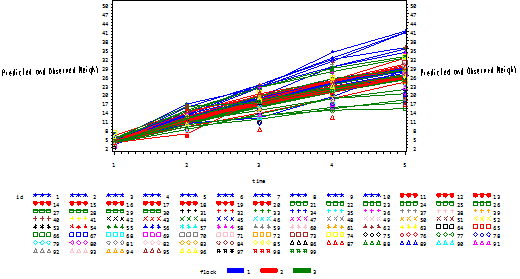Figure 6. Predicted values plotted against the actual values of body weight for the second model.

In the third model, an interaction of time*time*flock was included to indicate that the different flocks not only show different linear trends over time, but that they also show different quadratic trends over time, as shown in figure 7, 8. Results revealed that the effect of time*time*flock was significant (P< 0.05). Estimated criteria obtained that the third model was a better fit than the first and the second model (Table 7). Results showed that the effect of time*time*flock was significant (P=0.01) whereas the effect of flock was non-significant.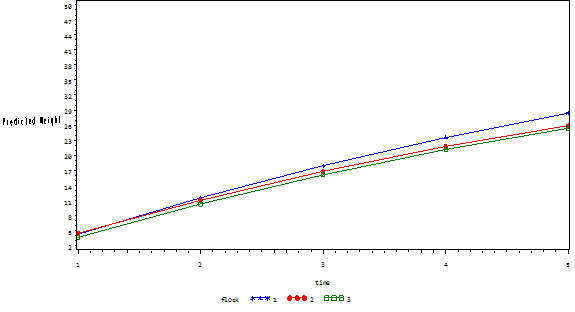Figure 7. Predicted values of the second model with the quadratic effect of time for the third model.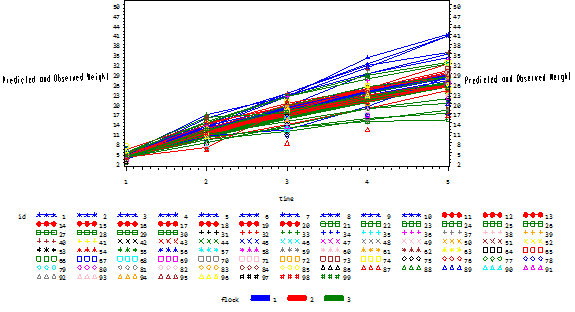Figure 8. Predicted values plotted against the actual values of body weight for the third model.

Table (10): showed that results of the third model. The effect of flock was not significant, whereas the other effects were significant. Solutions of fixed effects according to third model were shown in Table (11). In conclusion: it’s clear that in order to develop more effective and more powerful observational studies, mixed models methods should be used more systematically.

Table 10. Type 3 tests of fixed effects (Model 3).

 Effect DF Den DF F value P> F Time 1 96 1206.89 <0.0001 Flock 2 294 1.93 0.1476 Time*Flock 2 294 5.34 0.0053 Time*Time 1 96 140.60 <0.0001 Time*Time*Flock 2 294 4.37 0.0135

Table 11. Solution of fixed effects.

 Effect Estimate DF P Intercept -2.82±0.44 96 <0.0001 Time 7.02±0.36 96 <0.0001 Flock1 -1.30±0.66 294 0.0509 Flock2 -0.62±0.66 294 0.3481 Flock3 0 . . Time*Flock1 1.72±0.55 294 0.0020 Time*Flock2 1.23±0.55 294 0.0270 Time*Flock3 0 . . Time*Time -0.26±0.05 96 <0.0001 Time*Time*Flock1 -0.18±0.08 294 0.0225 Time*Time*Flock2 -0.21±0.08 294 0.0079 Time*Time*Flock3 0 . .

Mixed models provide powerful tests of repeated measurements effects. The mixed-model approach also enables researchers to make a comparison among different linear trends over time and different quadratic trends over time. Furthermore, numerical results can easily be obtained with several programs such as SAS. In view of these facts, it is obvious that SAS is efficient software. This software does not only provide estimates of the parameters in mixed models, but it also supplies the user with fit statistics and tests the significances.

Appendix1

data z;

input id flock time1 time2 time3 time4 time5;

cards;

1 1      4.00        12.00      18.00      22.5        27.0

2 1      3.50        13.00      19.00      29.0        35.0

3 1      4.50        16.50      22.00      34.0        41.0

4 1      3.00        11.50      19.00      24.0        27.5

5 1      3.50        10.00      12.00      19.0        28.0

6 1      5.00        12.50      22.00      31.0        40.5

7 1      5.00        13.50      23.00      32.0        40.5

8 1      5.00        13.00      22.50      29.0        34.0

9 1      3.50        12.50      18.50      25.0        28.5

10 1    4.00        15.50      23.00      31.5        35.5

. .         .              .              .              .              .

. .         .              .              .              .              .

. .         .              .              .              .              .

99 1    4.25        12.00      17.50      21.0        24.5

proc glm data=z;

class flock;

model time1 time2 time3 time4 time5= flock;

repeated time 5 ;

lsmeans flock /pdiff out=means;

run;

proc print data=means;

run;

goptions reset=all;

symbol1 c=blue v=star h=.8 i=j;

symbol2 c=red v=dot h=.8 i=j;

symbol3 c=green v=dot h=.8 i=j;

axis1 label=(a=4 'Means');

axis2 label=('Time') value=('1' '2' '3' '4' '5');

proc gplot data=means;

plot lsmean*_name_=flock/ vaxis=axis1 haxis=axis2;

run;

proc glm data=z;

class flock;

model time1-time5=flock; / nouni;

repeated w 5 / printe;

run;

Appendix 2

Reshape the data from its wide form to a long form.

proc transpose data=z out=long;

run;

proc transpose data=z out=long;

by id flock;

run;

data long;

set long (rename=(col1=weight) );

time = substr(_NAME_, 5, 1 )+0;

drop _name_;

run;

proc print data=long (obs=20);

var id flock time weight;

run;

proc print;

run;

proc sort data=long;

by id time;

run;

goptions reset=all;

symbol1 c=blue v=star h=.8 i=j r=10;

symbol2 c=red v=dot h=.8 i=j r=10;

symbol3 c=green v=square h=.8 i=j r=10;

axis1 order=(2 to 50 by 3) label=(a=2 'Weight');

proc gplot data=long;

plot weight*time=id / vaxis=axis1;

run;

proc mixed data=long covtest noclprint;

class id flock;

model weight = time flock time*flock / solution outp=pred1r outpm = pred1f;

random intercept time / subject = id;

run;

goptions reset=all;

symbol1 c=blue v=star h=.8 i=j;

symbol2 c=red v=dot h=.8 i=j;

symbol3 c=green v=square h=.8 i=j;

axis1 order=(2 to 50 by 3) label=(a=2 'Predicted Weight');

proc gplot data=pred1f;

plot pred*time=flock /vaxis=axis1;

run;

quit;

proc sort data=pred1f;

by time;

run;

goptions reset=all;

symbol1 c=blue v=star h=.8 i=j w=10;

symbol2 c=red v=dot h=.8 i=j w=10;

symbol3 c=green v=square h=.8 i=j w=10;

symbol4 c=blue v=star h=.8 i=j r=10;

symbol5 c=red v=dot h=.8 i=j r=10;

symbol6 c=green v=square h=.8 i=j r=10;

axis1 order=(2 to 50 by 3) label=(a=2 'Predicted and Observed Weight');

proc gplot data=pred1f;

plot pred*time=flock / vaxis=axis1 ;

plot2 weight*time = id / vaxis=axis1 ;;

run;

quit;

proc mixed data=long covtest noclprint;

class id flock;

model weight = time flock time*flock time*time / solution outp=pred2r outpm=pred2f ;

random intercept time / subject = id;

run;

proc sort data=pred2f;

by time;

run;

goptions reset=all;

symbol1 c=blue v=star h=.8 i=j ;

symbol2 c=red v=dot h=.8 i=j ;

symbol3 c=green v=square h=.8 i=j ;

axis1 order=(2 to 50 by 3) label=(a=2 'Predicted Weight');

proc gplot data=pred2f;

plot pred*time=flock /vaxis=axis1 ;

run;

quit;

proc sort data=pred2f;

by time;

run;

goptions reset=all;

symbol1 c=blue v=star h=.8 i=j w=10;

symbol2 c=red v=dot h=.8 i=j w=10;

symbol3 c=green v=square h=.8 i=j w=10;

symbol4 c=blue v=star h=.8 i=j r=10;

symbol5 c=red v=dot h=.8 i=j r=10;

symbol6 c=green v=square h=.8 i=j r=10;

axis1 order=(2 to 50 by 3) label=(a=2 'Predicted and Observed Weight');

proc gplot data=pred2f;

plot pred*time=flock / vaxis=axis1 ;

plot2 weight*time = id / vaxis=axis1 ;;

run;

proc mixed data=long covtest noclprint;

class id flock;

model weight = time flock time*flock time*time time*time*flock / solution outp=pred3r outpm=pred3f ;

random intercept time / subject = id;

run;

proc sort data=pred3f;

by time;

run;

goptions reset=all;

symbol1 c=blue v=star h=.8 i=j ;

symbol2 c=red v=dot h=.8 i=j ;

symbol3 c=green v=square h=.8 i=j ;

axis1 order=(2 to 50 by 3) label=(a=2 'Predicted Weight');

proc gplot data=pred2f;

plot pred*time=flock /vaxis=axis1 ;

run;

proc sort data=pred3f;

by time;

run;

goptions reset=all;

symbol1 c=blue v=star h=.8 i=j w=10;

symbol2 c=red v=dot h=.8 i=j w=10;

symbol3 c=green v=square h=.8 i=j w=10;

symbol4 c=blue v=star h=.8 i=j r=10;

symbol5 c=red v=dot h=.8 i=j r=10;

symbol6 c=green v=square h=.8 i=j r=10;

axis1 order=(2 to 50 by 3) label=(a=2 'Predicted and Observed Weight');

proc gplot data=pred3f;

plot pred*time=flock / vaxis=axis1 ;

plot2 weight*time = id / vaxis=axis1 ;;

run;

References

1. Akbaş Y., Fırat M.Z., and Yakupoğlu C. 2001. Comparison of different models used in the analysis of repeated measurements in animal science and their SAS applications. Agricultural Information Technology Symposium, Sütçü İmam University, Agricultural Faculty, Kahramanmaraş, 20-22 September.
2. Algina J., and Kesselman H.J. 1997. Detecting repeated measures effects with univariate and multivariate statistics. Psychological Methods., 2: 208-218.
3. Eyduran E., Tatliyer A., Waheed, A., Tariq M. M. 2013. Determination of the most appropriate covariance structure for data with missing observations in repeated measures design., Ksu. J. Nat. Sci., 16(3): 32-37.
4. Eyduran E., and Akbaş Y. 2010. Comparison of diıfferent covariance structure used for experimental design with repeated measurement. J. Anim. Plant Sci., 20(1): 44-51.
5. Ganesan R., Dhanavanthan P., Kiruthika C., Kumarasamy P., and Balasubramanyam D. 2014. Comparative study of linear mixed-effects and artificial neural network models for longitudinal unbalanced growth data of Madras Red sheep. Veterinary World., 7(1): 52 – 58.
6. Greenhouse S. W., and Geisser S. 1959. On methods in the analysis of profile data. Psychometrika., 24: 95-112.
7. Huynh H., and Feldt L. 1970. Conditions under which mean square ratios in repeated measurements designs have exact F distributions. Journal of the American Statistical Association, 65: 1582-1589.
8. Huynh H., and Feldt L. S. 1976. Estimation of the Box correction for degrees of freedom from sample data in randomized block and split-plot designs. Journal of Educational Statistics, 1: 69-82.
9. Kebede K., and Gebretsadik G. 2010.Statistical modeling of growth performance data on sheep using mixed linear models. Livestock Research for Rural Development.Volume 22, Article #80.
10. Keskin S., and Mendeş M. 2001. Experimental Designs including repeated measurement in one’s levels of their factors. S.Ü. J. Agricultural Faculty.,15(25): 42-53.
11. Lal K. 2010. Analysis of repeated measures data using SAS. Indian Agricultural Statistics Research Institute. Library Avenue, Pusa, New Delhi.,113-120.
12. Little R. C., Henry P. R., and Ammerman C. B. 1998. Statistical analysis of repeated measures data using SAS procedures. J. Anim. Sci., 76:1216-1231.http://jas.fass.org/cgi/reprint/76/4/1216.
13. Littell R. C., Milliken G. A., Stroup W. W., and Wolfinger R. D. 1996. SAS system for mixed models, Cary, NC: SAS Institute.
14. Orhan H., Eyduran E., Akbaş Y. 2010. Defining The Best Covariance Structure for Sequential Variation on Live Weights of Aanatolian Merinos Male Lambs. J. Anim. and Plant Sci., 20(3), 158-163.
15. SAS Institute. 2010. SAS / STAT Users Guide for Personal Computer. Release 9.1, 2010; Inc., Cary, N.C., USA.

 Contents 1. 2. 3. 1 2
Article ToolsAbstractPDF(382K)